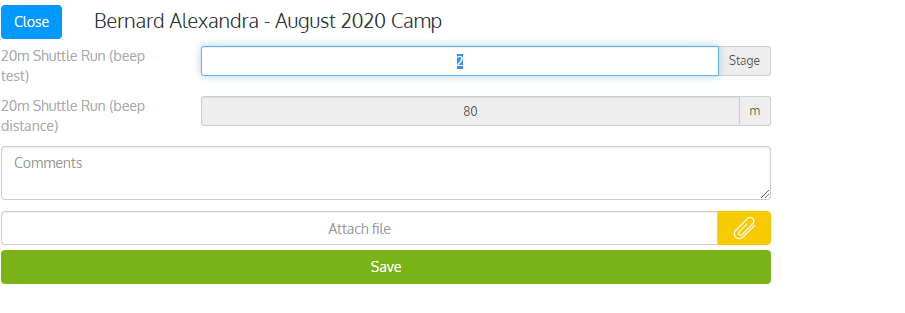It is possible to use IF THEN logic in assessment and custom forms formulas. There is no direct IF THEN syntax but the same result can be achieved by using the comparison operators below.

> (greater than)
< (less than)
<= (less than or equal)
>= (greater than or equal)
== (equal)

### Example

The example below illustrates an IF THEN formula used to return total distance covered during a shuttle run test. The recorded data is the last completed stage. The calculated result will be the total distance covered at the end of the last completed stage.

Formula: (laststa==1)(40)+(laststa==2)(80)+(laststa==3)(120)

laststa= last completed stage

== comparison operator

(40), (80), (120)=returned value when the condition laststa ==  is met

Each block condition  and return value (eg: (laststa==1)(40) must be separated by a + sign. You can add as many block as you need and use a combination of comparison operators

To use this formula, you'll need to create a 'Shuttle test (distance)' that will be the result of the formula.Then add the new test to the batteryAnd finally enter data and click Save. The distance covered will be automatically calculated.You can use the same logic/syntax to add any IF THEN formula. For example, to return an age and gender related predicted VO2max.

(gender==1)*((age<30)*53+and(age>=30,age<40)*50+and(age>=40,age<50)*45+and(age>=50,age<60)*43+(age>=60)41)(gender==0)((age<30)*33+and(age>=30,age<40)*30+and(age>=40,age<50)*27+and(age>=50,age<60)*24(age>=60)*23)